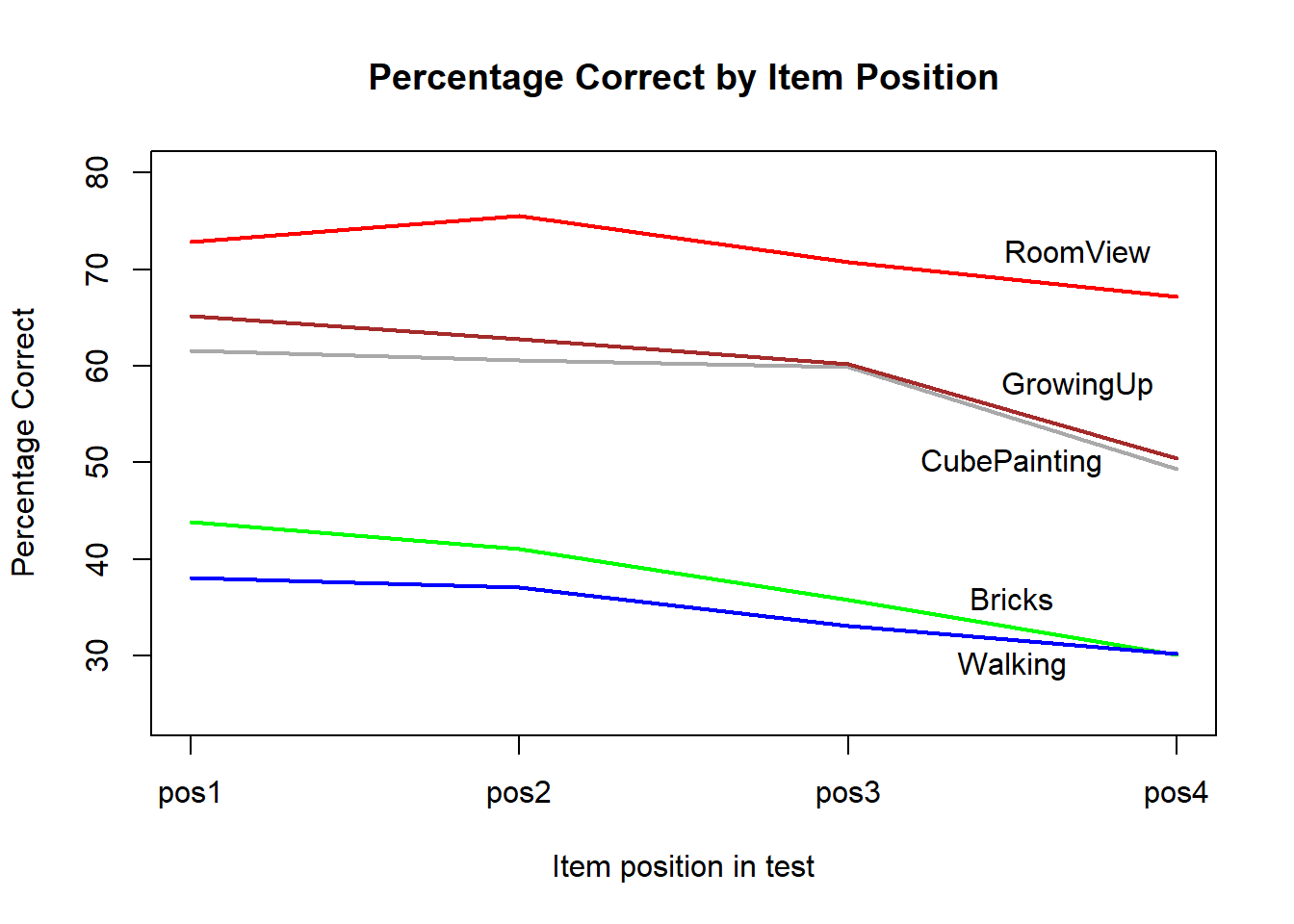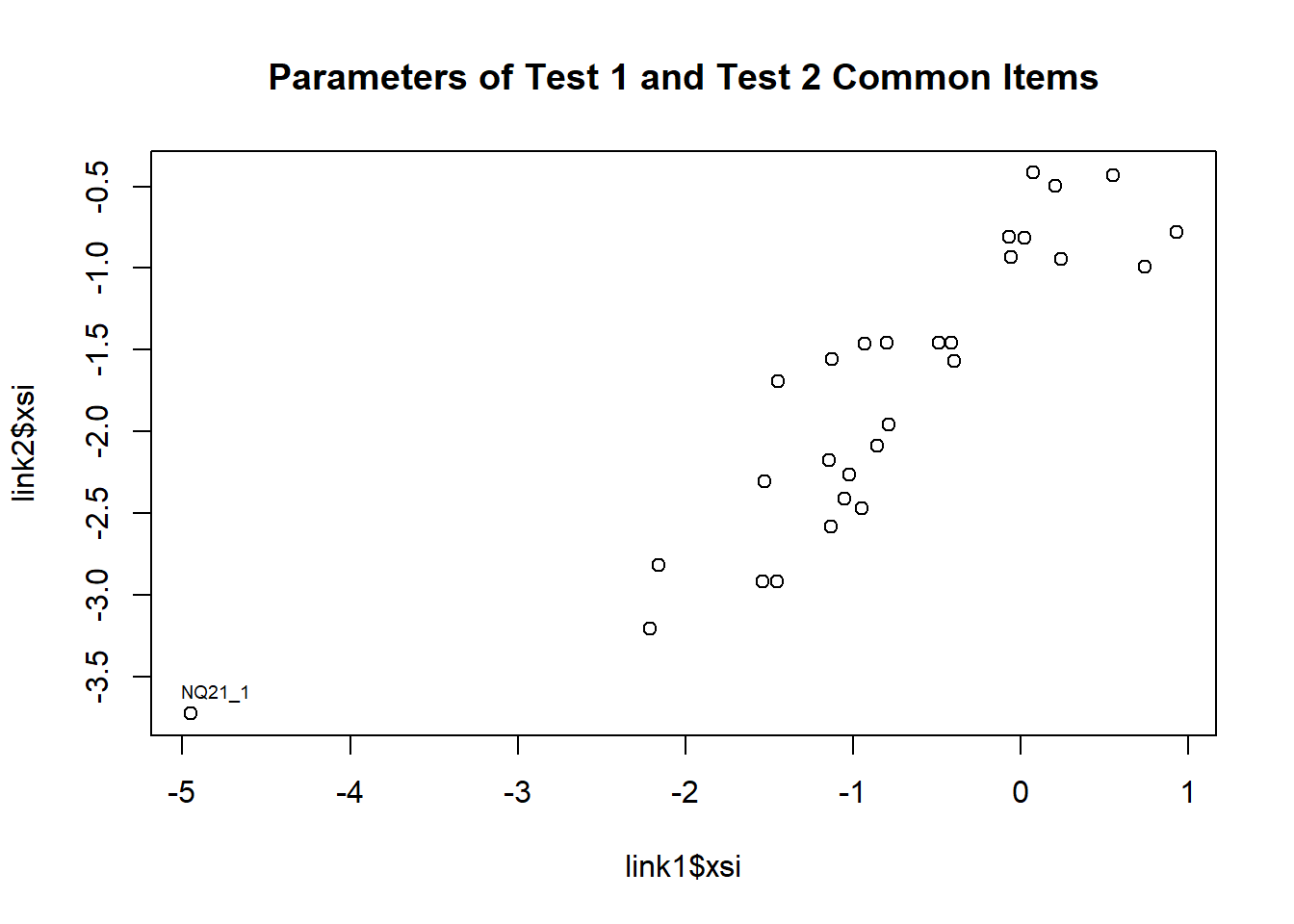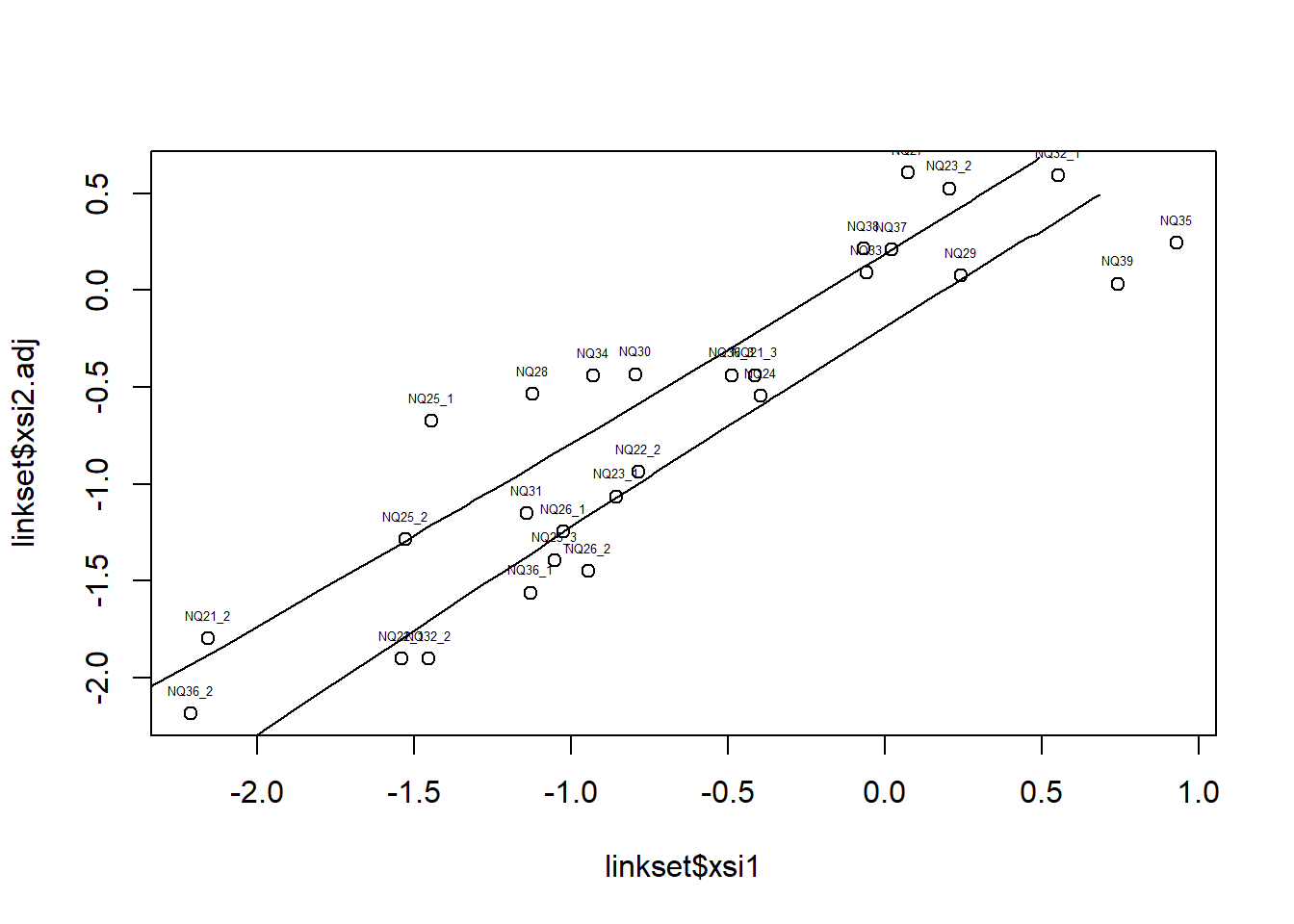Chapter 9 Equating Tests. Part 2: Practice

In this session, we will learn about
- practical considerations in equating tests
- checking item invariance across different tests
- writing user-defined functions in R.

9.1 Differences between Theory and Practice

In theory, the concept of equating is simple: there needs to be something in common between two tests, either by common items or common persons. One needs to align the two tests. While there are different methods to place two tests on the same scale, all the methods should produce the same results, provided that the data fit the underlying mathematical model.

In practice, however, the data rarely fit the model. An important assumption in equating tests is that the common items are “invariant” across the two tests. That is, an item has the same difficulty when it is placed in both Test 1 and Test 2. If the difficulty of an item changes when it is placed in different tests, then that item is essentially a different item, and it cannot be used to equate two tests.

There are many reasons for why an item may have different difficulties when placed in two different tests. We will look at two particular factors: item position effect and differential item functioning.

9.2 Item Position Effect

It has been observed that the position of the placement of an item in a test affects the item’s difficulty. An item placed at the beginning of a test appears to be easier than the same item placed at the end of a test. We will call this the fatigue effect. This effect has been observed in the PISA tests. In PISA 2003, test items were placed according to a rotated test booklet design. Each test booklet was divided into four sections. Each item appeared in four test booklets, in each of the four sections. The test booklets were randomly distributed to students, so it could be assumed that the average ability of students taking each booklet was the same across all booklets.

The following shows five PISA 2003 mathematics items, and the percentages correct when the items were placed in each of the four sections of a test.

Table 9.1: Percentage Correct by Item Position
RoomView Bricks Walking CubePainting GrowingUp
pos1 72.9 43.9 38.1 61.6 65.2
pos2 75.6 41.1 37.1 60.6 62.8
pos3 70.8 35.8 33.1 59.9 60.2
pos4 67.2 30.1 30.2 49.3 50.4

Reading down the columns of Table 9.1, you can see that the percentage correct generally decreases from position 1 to position 4. The percentage correct was the lowest when an item was placed at the end of the test (position 4). Graphically, we can see this trend in Figure 9.1.Figure 9.1: Percentages correct of five items at four positions of a test

The implication of item position effect is that if one item is placed at the beginning of one test and at the end of another test, then the item cannot be regarded as the same item because their difficulties are different, even though the wording of the item has not changed. The presence of item position effect threatens the equating process.

9.3 Differential Item Functioning

Differential item functioning (typically abbreviated as DIF) is present when the probability of success on an item differs for two groups of people, even when we control for ability measures. For example, in mathematics, girls have been found to outperform boys on number items, while boys outperform girls on spatial items, even when the average abilities for boys and girls are the same. Thus if we have many spatial items as common items linking two tests, the result of the equating may be distorted. Differential item functioning may occur because of geolocations, gender, SES, curriculum and many other factors.

9.4 Minimise Violations of Item Invariance Assumptions

There are several ways to mitigate threats to equating due to model violations:
- Use a large number of common items.
- Check for item invariance and remove common items that are not invariant.
- Use a balanced rotated test design for the placement of test items.

With regards to the number of common items required for equating, it is difficult to put a figure to it. We can say that more than 30 common items are needed, judging from our experience.

9.5 Checking for Item Invariance

The following shows a procedure for checking for item invariance.

9.5.1 Separately scale each test

Using the data sets N1.csv, N2.csv and N1N2Specs.docx that we used in Section 8.6. Scale each test separately and obtain item parameters for each test.

rm(list=ls())

# Make sure you change the path to the data set in the two commands below.
stringsAsFactors = FALSE, colClasses = rep("character", 50))
stringsAsFactors = FALSE, colClasses = rep("character", 50))

key1 <- "32213211431123114141111143411111211312111111323121"
key2 <- "11143411111211312111111323121414324231413131111242"

key1 <- unlist(strsplit(key1,""))
key2 <- unlist(strsplit(key2,""))

library(CTT)

s1 <- score(N1,key1,output.scored = TRUE)
s2 <- score(N2,key2,output.scored = TRUE)

resp1 <- s1$scored resp2 <- s2$scored

library(TAM)
mod1 <- tam.jml(resp1)
mod2 <- tam.jml(resp2)

#Check item statistics
tctt1 <- tam.ctt(resp1,mod1$WLE) tctt2 <- tam.ctt(resp2,mod1$WLE)

Find common items between the two tests.

# Find common items between N1 and N2
common1 <- mod1$item1$xsi.label %in% mod2$item1$xsi.label
link1 <- mod1$item1[common1, ] common2 <- match(link1$xsi.label,mod2$item1$xsi.label)
link2 <- mod2$item1[common2, ] Variables link1 and link2 only contain the common items. Plot the item parameters for both tests to see the extent of agreement. plot(link1$xsi,link2xsi, main="Parameters of Test 1 and Test 2 Common Items")Figure 9.2: Plot of common item parameters for Test 1 and Test 2 One item, NQ21_1 (the first link item), appears to be a clear outlier in this set. It is by far the easiest item, and the standard error will tend to be large. Let us remove this item from the common item set. link1 <- link1[-1,] #take away the first link item link2 <- link2[-1,] #take away the first link item We can see in Figure 9.2 that while there is a general positive correlation between the two sets of item parameters, the scales are not the same. Test 1 common item parameters (without the first link item) range between -2.21 and 0.93, while Test 2 parameters range between -3.21 and -0.42. We will need to compute a shift for the parameters of Test 2 so the two sets of parameters will align with the same mean value. # Adjust Test 2 common items to have the same mean as the mean of Test 1 common items xsi1 <- link1xsi
xsi2 <- link2$xsi shift <- mean(xsi1) - mean(xsi2) xsi2.adj <- xsi2 + shift The shift is 1.0216. Place the Test 1 and Test 2 common item parameters in one data frame, and sort the items in order of the (absolute) magnitude of the differences between pairs of item parameters. # Compute the difference between pairs of common item parameters diff <- xsi1 - xsi2.adj linkset <- data.frame(xsi1, xsi2, xsi2.adj, diff, link1$se.xsi, link2$se.xsi) colnames(linkset) <- c("xsi1","xsi2","xsi2.adj","diff", "se1", "se2") rownames(linkset) <- link1$xsi.label
library(knitr)
kable(linkset,digits = 3,align="ccccc", caption="Differences in Test 1 and Test 2 Item Parameters ")
Table 9.2: Differences in Test 1 and Test 2 Item Parameters
xsi1 xsi2 xsi2.adj diff se1 se2
NQ31 -1.142 -2.174 -1.152 0.011 0.078 0.078
NQ21_3 -0.416 -1.460 -0.438 0.022 0.073 0.066
NQ36_2 -2.214 -3.206 -2.184 -0.030 0.098 0.112
NQ32_1 0.551 -0.430 0.592 -0.041 0.074 0.057
NQ36_3 -0.488 -1.460 -0.438 -0.050 0.073 0.066
NQ33 -0.059 -0.932 0.089 -0.148 0.072 0.060
NQ24 -0.395 -1.568 -0.546 0.151 0.072 0.067
NQ22_2 -0.785 -1.959 -0.937 0.152 0.074 0.074
NQ29 0.242 -0.946 0.075 0.167 0.072 0.060
NQ37 0.022 -0.814 0.207 -0.185 0.072 0.059
NQ23_1 -0.856 -2.086 -1.064 0.208 0.075 0.076
NQ26_1 -1.025 -2.267 -1.245 0.220 0.077 0.081
NQ25_2 -1.527 -2.305 -1.284 -0.243 0.083 0.081
NQ38 -0.069 -0.807 0.214 -0.283 0.072 0.059
NQ23_2 0.206 -0.497 0.525 -0.319 0.072 0.057
NQ25_3 -1.054 -2.413 -1.391 0.338 0.077 0.084
NQ22_1 -1.541 -2.922 -1.900 0.359 0.083 0.100
NQ30 -0.796 -1.456 -0.434 -0.362 0.075 0.066
NQ21_2 -2.158 -2.817 -1.796 -0.362 0.097 0.097
NQ36_1 -1.130 -2.582 -1.560 0.430 0.078 0.089
NQ32_2 -1.454 -2.922 -1.900 0.446 0.082 0.100
NQ34 -0.929 -1.464 -0.443 -0.486 0.076 0.066
NQ26_2 -0.946 -2.470 -1.448 0.503 0.076 0.086
NQ27 0.073 -0.417 0.605 -0.532 0.072 0.057
NQ28 -1.124 -1.559 -0.537 -0.587 0.078 0.067
NQ35 0.930 -0.777 0.245 0.685 0.077 0.059
NQ39 0.742 -0.989 0.033 0.709 0.075 0.060
NQ25_1 -1.447 -1.695 -0.673 -0.774 0.082 0.069

In Table 9.2, it can be seen that several common items have very different item difficulty measures in Test 1 and Test 2. For example, the difference in item parameter estimates for Item NQ25_1 and for Item NQ39 is larger than 0.7 logit. These items are clearly not suitable as common items for equating purposes.

It is clearer if we plot the item estimates with a confidence band, and examine the items visually. To calculate the confidence band, we will use the formulation in Rating Scale Analysis , p115-116.

First, for each item, we compute the average of the two item estimates for the two tests, say, $$d.=(xsi_1 + xsi.adj_2)/2$$. Then, we compute the standard error of this average, $$s=\frac{1}{2}sqrt(s_1^2+s_2^2)$$ where $$s_1$$ and $$s_2$$ are the standard errors of the two item parameter estimates respectively. For the 95% confidence band, we compute $$p_1=d.-2\times s$$ and $$p_2=d.+2\times s$$. Graphically, we use the points $$(p_2, p_1)$$ for the lower bound of the confidence band, and the points $$(p_1, p_2)$$ for the upper bound, for all the items. The following R code shows this procedure, where we create an R function to do this plotting.

d <- (linkset$xsi1 + linkset$xsi2.adj)/2
s <- (1/2)*sqrt(linkset$se1^2 + linkset$se2^2)
p1 <- d - 2*s
p2 <- d + 2*s

plot(linkset$xsi1, linkset$xsi2.adj)
lines(p1[order(p1)],p2[order(p1)])
lines(p2[order(p1)],p1[order(p1)])
text(linkset$xsi1,linkset$xsi2.adj,labels=rownames(linkset),cex=0.4,pos=3)
}Figure 9.3: Comparing Two Sets of Item Paramters

From Figure 9.3 and Table 9.2, we may need to remove some items as common items, as some items do not seem to have invariance property when placed in two different tests. However, it is not an exact science to decide on the list of items to remove, and there is no standard procedure to follow. Generally, this is a trial-and-error process. Perhaps it will be best if several researchers independently make selections of common items and compare notes afterwards.

When selecting (or removing) common items, there are a few things to keep in mind.
- The higher the number of common items, the more reliable the equating process will be. Sure, one can choose two common items, and they will fall perfectly on one line with no outliers. But the problem is that any pair of items will lead to vastly different equating shift, so we won’t know which pair is the best. When there are many common items, the impact of an outlier will be less significant.
- When some common item are removed, the mean values of the remaining set will change, so an item that was an outlier may fall within the confidence band. Generally, the sequence of removing common items will have an impact on which items become outliers. As an example, we remove items NQ25_1, NQ28, NQ34, NQ30. We will write a function for re-calculating the shift and the adjusted Test 2 item parameters after removing some common items.

shift <-  mean(linkset2$xsi1) - mean(linkset2$xsi2)
xsi2.adj <- linkset2$xsi2 + shift diff <- linkset2$xsi1 - xsi2.adj
linkset2$xsi2.adj <- xsi2.adj linkset2$diff <- diff
}
remItems <- c("NQ25_1","NQ28","NQ34","NQ30")